# How to plot the coherence between two signals in Python?

• Difficulty Level : Easy
• Last Updated : 15 May, 2020

Matplotlib is an amazing visualization library in Python for 2D plots of arrays. Matplotlib is a multi-platform data visualization library built on NumPy arrays and designed to work with the broader SciPy stack.

## What is Coherence and and Correlation?

Coherence: It is used for measuring the correlation between two signals.
Correlation : It defines the degree of dependency of one quantity over the other. If one quantity is totally dependent on other then the correlation between them is said to be 1. If two quantities or variables are not related to each other then they have zero correlation.
Coherence is the normalized cross-spectral density:

Attention geek! Strengthen your foundations with the Python Programming Foundation Course and learn the basics.

To begin with, your interview preparations Enhance your Data Structures concepts with the Python DS Course. And to begin with your Machine Learning Journey, join the Machine Learning - Basic Level CourseIn Python, Matplotlib.pyplot.cohere() is used to find the coherence between two signals.

Syntax: matplotlib.pyplot.cohere(x, y, NFFT=256, Fs=2, Fc=0, detrend=, window=, noverlap=0, pad_to=None, sides=’default’, scale_by_freq=None, *, data=None, **kwargs)

Parameters: This method accepts the following parameters-
1)x, y : It is the sequence of data.
2) Fs: It is a scalar parameter and its default value is 2,
3) window: This parameter takes a data segment as an argument and returns the windowed version of the segment. Its default value is window_hanning()
4) sides: This parameter specifies which sides of the spectrum to return. This can have the following values: ‘default’, ‘one-sided’, and ‘two-sided’.
5) pad_to : This parameter contains the integer value to which the data segment is padded.
6) Fc: This parameter also contains the integer value to offsets the x extents of the plot to reflect the frequency range. Its default value is 0
7) NFFT: This parameter contains the number of data points used in each block for the FFT.
8) detrend: This parameter contains the function applied to each segment before fft-ing, designed to remove the mean or linear trend {‘none’, ‘mean’, ‘linear’}.
scale by freq: This parameter allows for integration over the returned frequency values.
9) overlap : This parameter is the number of points of overlap between blocks.
10) Fc : This parameter is the center frequency of x.

Returns : This method returns the following-
1) Cxy: This returns the coherence vector.
2) freqs: This returns the frequencies for the elements in Cxy.

The resultant is (Cxy, freqs)

Let’s see the below examples to where we will find the coherence between the two signals using the above function.

Example 1:

 import numpy as npimport matplotlib.pyplot as plt    # signal 1time1=np.arange(0,100,0.1)cossignal1= np.cos(time1)  plt.plot(cossignal1)plt.title("Signal 1")plt.show()    # signal 2time2=np.arange(0,100,0.1)cossignal2= np.cos(time2)  plt.plot(cossignal2)plt.title("Signal 2")plt.show()  # Store the value of correlation in a# variable say 'cor' using the following code:cor=plt.cohere(cossignal1,cossignal2)    # plot the coherence graphplt.show()

Output: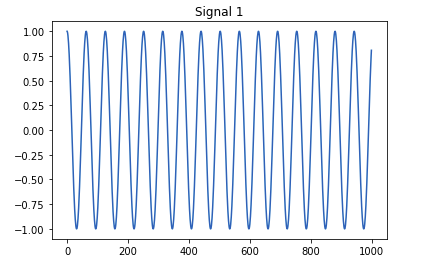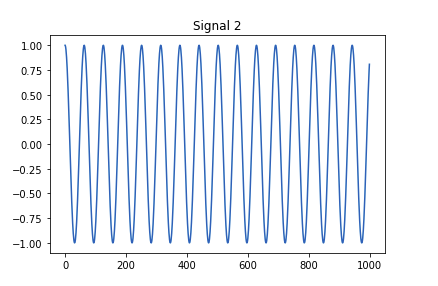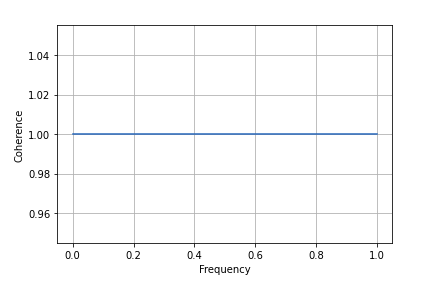Example 2: Coherence between sine and cosine signal

 import numpy as npimport matplotlib.pyplot as plt    # signal 1time1 = np.arange(0, 100, 0.1)sinsignal1 = np.sin(time1)  plt.plot(sinsignal1)plt.title("Sine Signal")plt.show()  # signal 2time2 = np.arange(0, 100, 0.1)cossignal2 = np.cos(time2)  plt.plot(cossignal2)plt.title("Cose Signal")plt.show()  # Store the value of correlation in# a variable say 'cor' using the # following codecor = plt.cohere(sinsignal1, cossignal2)      # Plot the coherence graphplt.show()

Output: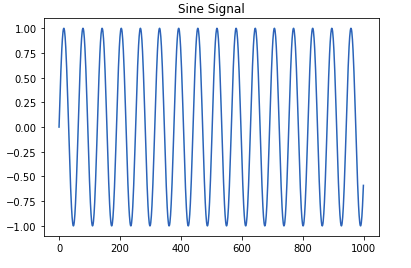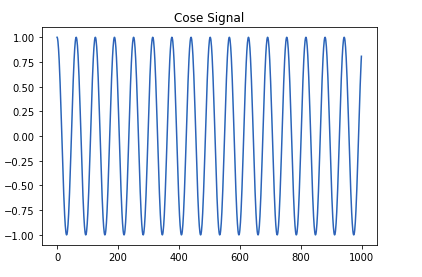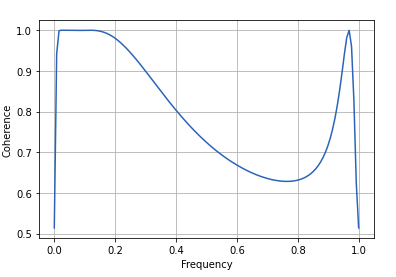My Personal Notes arrow_drop_up